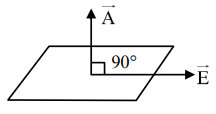# Choose the incorrect statement:Question:

Choose the incorrect statement:

(a) The electric lines of force entering into a Gaussian surface provide negative flux.

(b) A charge ' $q$ ' is placed at the centre of a cube. The flux through all the faces will be the same.

(c) In a uniform electric field net flux through a closed Gaussian surface containing no net charge, is zero.

(d) When electric field is parallel to a Gaussian surface, it provides a finite non-zero flux.

Choose the most appropriate answer from the options given below

1. (c) and (d) only

2. (b) and $(d)$ only

3. (d) only

4. (a) and (c) only

Correct Option: , 3

Solution:

Since $\phi=\overrightarrow{\mathrm{E}} \cdot \overrightarrow{\mathrm{A}}=\mathrm{EA} \cos \theta$$\theta=90^{\circ}$

$\therefore \phi=0$